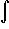#Interactive Real Analysis

Next | Previous | Glossary | Map

## 7.4. Lebesgue Integral

### Example 7.4.6(b): Lebesgue Integral for Bounded Functions

Is the function f(x) = x2 Lebesgue integrable over the rational numbers inside [0, 2]? If so, find the integral.
Let Q be the rational numbers inside [0, 2] and define the functions
s(x) = 0
S(x) = 4 XQ(x)

Then s(x)f(x)S(x) over Q, and both s and S are simple functions. Therefore

I*(f)LS(x) dx = 4 m(Q) = 0
and
I*(f)Ls(x) dx = 0
Since also I*(f)LI*(f)L we have that I*(f)L = I*(f)L = 0. Therefore the function is integrable and the value of the integral is zero.
Next | Previous | Glossary | Map